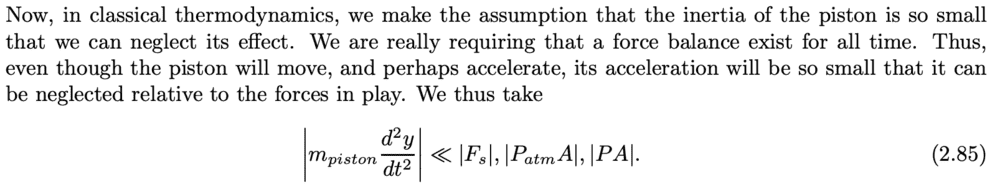# Ignoring the acceleration of a piston in thermodynamics

etotheipi
I was just reading a set of thermodynamics lecture notes and came across the followingIn most thermodynamics problems I have done, it is indeed assumed that the piston does not accelerate so we can simply equate forces on the piston. However, I don't fully understand the line of reasoning given above.

For instance, if the pressure of the gas inside a frictionless piston far exceeds that of the external gas, surely it would be detrimental to ignore the acceleration?

Chestermiller
Mentor
I was just reading a set of thermodynamics lecture notes and came across the following

View attachment 253810

In most thermodynamics problems I have done, it is indeed assumed that the piston does not accelerate so we can simply equate forces on the piston. However, I don't fully understand the line of reasoning given above.

For instance, if the pressure of the gas inside a frictionless piston far exceeds that of the external gas, surely it would be detrimental to ignore the acceleration?
I think it makes more sense to consider the case of a massless frictionless piston, in which case the forces acting on the two opposing faces are equal to one another. In any event, even if the piston has mass, if the system is in equilibrium at the final state, all the kinetic energy imparted to the piston during the process is recovered in the end when the piston is no longer moving.

•etotheipi
Chestermiller
Mentor
If we do a force balance on the piston, we obtain:
$$F_g-P_{ext}A=m_{piston}\frac{dv}{dt}$$where ##F_g## is the force exerted by the gas on the piston. If we multiply this equation by the piston velocity v and integrate, we obtain the mechanical energy balance on the piston:$$W_g-\int{P_{ext}dV}=KE=m_{piston}\frac{v^2}{2}$$In the final state of the system, when the piston has reached its equilibrium position, its velocity and kinetic energy are zero. Therefore, for the final equilibrium state, $$W_g=\int{P_{ext}dV}$$and the work done by the gas on its surroundings is just the volume integral of the external force.

•etotheipi
etotheipi
If we do a force balance on the piston, we obtain:
$$F_g-P_{ext}A=m_{piston}\frac{dv}{dt}$$where ##F_g## is the force exerted by the gas on the piston. If we multiply this equation by the piston velocity v and integrate, we obtain the mechanical energy balance on the piston:$$W_g-\int{P_{ext}dV}=KE=m_{piston}\frac{v^2}{2}$$In the final state of the system, when the piston has reached its equilibrium position, its velocity and kinetic energy are zero. Therefore, for the final equilibrium state, $$W_g=\int{P_{ext}dV}$$and the work done by the gas on its surroundings is just the volume integral of the external force.

Thank you, this is really useful. I also assume that by Newton III, since the gas and piston have the same velocity, the work done on the gas is ##-W_g##.

So if we add a friction term ##F_{f}##, we would then obtain $$F_g-P_{ext}A - F_{f}=m_{piston}\frac{dv}{dt}$$ which would consequently give $W_{g} = W_{f} + \int{P_{ext}dV}$ once equilibrium has been reached.

However, is it the case that if we want to analyse the system at a point where it has not yet reached equilibrium, the only correct route would be somehow integrate the internal pressure (excluding the case of the massless piston where this is always equal to the external pressure)?

Also, just as a sanity check, am I definitely correct in stating that the work done by the piston on the internal gas always equals the negative of the work done by the internal gas on the piston?

Chestermiller
Mentor
Thank you, this is really useful. I also assume that by Newton III, since the gas and piston have the same velocity, the work done on the gas is ##-W_g##.

So if we add a friction term ##F_{f}##, we would then obtain $$F_g-P_{ext}A - F_{f}=m_{piston}\frac{dv}{dt}$$ which would consequently give $W_{g} = W_{f} + \int{P_{ext}dV}$ once equilibrium has been reached.
Including piston friction is a little tricky, and one must be precise in specifying whether the system includes both the gas and the piston, or whether it includes only the gas. Both approaches, of course, will lead to the same final results, but the equations to reach these results will differ. For a more complete treatment specifically addressing piston friction, see this thread: https://www.physicsforums.com/threads/thermodynamics-gas-expansion-with-piston-friction.963282/
However, is it the case that if we want to analyse the system at a point where it has not yet reached equilibrium, the only correct route would be somehow integrate the internal pressure (excluding the case of the massless piston where this is always equal to the external pressure)?
To do it for the transient case (as a function of time), you would have to solve the compressible Navier Stokes viscous fluid equations (partial differential equations in space and time), in conjunction with the compressible continuity (mass balance) equation, and the differential thermal energy balance equation throughout the gas. If turbulence were present, this would be even more complicated.
Also, just as a sanity check, am I definitely correct in stating that the work done by the piston on the internal gas always equals the negative of the work done by the internal gas on the piston?
Yes. This is a consequence of Newton's 3rd law.